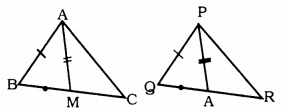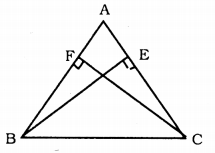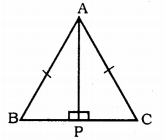# KSEEB Solutions for Class 9 Maths Chapter 5 Triangles Ex 5.3

KSEEB Solutions for Class 9 Maths Chapter 5 Triangles Ex 5.3 are part of KSEEB Solutions for Class 9 Maths. Here we have given Karnataka Board Class 9 Maths Chapter 5 Triangles Exercise 5.3.

## Karnataka Board Class 9 Maths Chapter 5 Triangles Ex 5.3

Question 1.
∆ABC and ∆DBC are two isosceles triangles on the same base BC and vertices A and D are on the same side of BC. If AD is extended to intersect BC at P, show that(i) ∆ABD ≅ ∆ACD
(ii) ∆ABP ≅ ∆ACP
(iii) AP bisects ∠A as well as ∠D.
(iv) AP is the perpendicular bisector of BC.
Solution:
Data : ∆ABC and ∆DBC are two isosceles triangles on the same base BC and vertices A and D are on the same side of BC. AD is extended to intersect BC at P.
To Prove:
(i) ∆ABD ≅ ∆ACD
(ii) ∆ABP ≅ ∆ACP
(iii) AP bisects ∠A as well as ∠D.
(iv) AP is the perpendicular bisector of BC
(v) AD is the angular bisector of ∠A.
Proof:
(i) In ∆ABD and ∆ACD,
AB = AC (data)
BD = DC (data)
S.S.S. Congruence rule.
∴ ∆ABD ≅ ∆ACD

(ii) In ∆ABP and ∆ACP,
AB = AC (data)
∠ABP = ∠ACP (Opposite angles)
∠BAP = ∠CAP (∵ ∆ABD ≅ ∆ACD proved)
Now ASA postulate.
∆ABP ≅ ∆ACP.

AP bisects ∠A.
In ∆BDP and ∆CDP,
BD = DC (data)
BP = PC (proved)
DP is common.
∴ ∆BDP ≅ ∆CDP (SSS postulate)
∴ ∠BDP = ∠CDP
∴ DP bisects ∠D.
∴ AP bisects ∠D.

(iv) Now, ∠APB + ∠APC = 180° (Linear pair)
∠APB + ∠APB = 180°
2 ∠APB = 180
∴ ∠APB = $$\frac{180}{2}$$
∴∠APB = 90°
∠APB = ∠APC = 90°
BP = PC (proved)
∴ AP is the perpendicular bisector BC.

(v) AP is the angular bisector of ∠A.
Angular bisector of ∠A is aD, because AD, AP is in one line.

Question 2.
AD is an altitude of an isosceles triangle ABC in which AB = AC. Show that ASolution:
Data: AD is an altitude of an isosceles triangle ABC in which AB = AC.
To Prove:
Proof: i) In ∆ABD and ∆ACD,
AB = AC (data)
∴ ∆ABD ≅ ∆ACD
∴ BD = DC

Question 3.
Two sides AB and BC and median AM of one triangle ABC are respectively equal to sides PQ and QR and median PN of ∆PQR. Show that :(i) ∆ABM ≅ ∆PQN
(ii) ∆ABC ≅ ∆PQR.
Solution:
Data: Two sides AB and BC and median AM of one triangle ABC are respectively equal to sides PQ and QR and median PN of ∆PQR.
To Prove:
(i) ∆ABM ≅ ∆PQN
(ii) ∆ABC ≅ ∆PQR.
Proof: (i) In ∆ABC,
AM is the median drawn to BC.
∴ BM = $$\frac{1}{2}$$ BC
Similarly, in ∆PQR,
QN = $$\frac{1}{2}$$ QR
But, BC = QR
$$\frac{1}{2}$$ BC = $$\frac{1}{2}$$ QR
∴ BM = QN
In ∆ABM and ∆PQN,
AB = PQ (data)
BM = QN (data)
AM = PN (proved)
∴ ∆ABM ≅ ∆PQN (SSS postulate)

(ii) In ∆ABC and ∆PQR,
AB = PQ (data)
∠ABC = ∠PQR (proved)
BC = QR (data)
∴ ∆ABC ≅ ∆PQR (SSS postulate)

Question 4.
BE and CF are two equal altitudes of a triangle ABC. Using the RHS congruence rule, prove that the triangle ABC is isosceles.Solution:
Data: BE and CF are two equal altitudes of a triangle ABC.
To Prove: ABC is an isosceles triangle.
Proof : BE = CF (data)
In ∆BCF and ∆CBE,
∠BFC = ∠CEB = 90° (data)
BC is a common hypotenuse.
As per Right angle, hypotenuse, side postulate,
∴ ∆BCF ≅ ∆CBE
∴ ∠CBF = ∠BCE
∴ ∠CBA = ∠BCA
∴ AB = AC
∴ ∆ABC is an isosceles triangle.

Question 5.
ABC is an isosceles triangle with AB = AC. Draw AP ⊥ BC to show that ∠B = ∠C.Solution:
Data: ABC is an isosceles triangle with AB = AC.
To Prove : ∠B = ∠C
Construction: Draw AP ⊥ BC.
Proof: In ∆ABC, AP ⊥ BC and AB = BC.
∴ In ∆ABP and ∆ACP
∠APB = ∠APC = 90° ( ∵ AP ⊥ BC)
Hypotenuse AB = Hypotenuse AC
AP is common.
As per RHS Postulate,
∆ABP ≅ ∆ACP
∴ ∠ABP = ∠ACP
∴ ∠ABC = ∠ACB
∴∠B = ∠C.

We hope the KSEEB Solutions for Class 9 Maths Chapter 5 Triangles Ex 5.3 helps you. If you have any query regarding Karnataka Board Class 9 Maths Chapter 5 Triangles Exercise 5.3, drop a comment below and we will get back to you at the earliest.

error: Content is protected !!